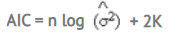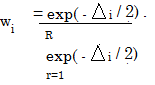# Akaike’s Information Criterion: Definition, Formulas

Share on

Parsimonious Model > Akaike’s Information Criterion

## What is Akaike’s Information Criterion?

Akaike’s information criterion (AIC) compares the quality of a set of statistical models to each other. For example, you might be interested in what variables contribute to low socioeconomic status and how the variables contribute to that status. Let’s say you create several regression models for various factors like education, family size, or disability status; The AIC will take each model and rank them from best to worst. The “best” model will be the one that neither under-fits nor over-fits.

Although the AIC will choose the best model from a set, it won’t say anything about absolute quality. In other words, if all of your models are poor, it will choose the best of a bad bunch. Therefore, once you have selected the best model, consider running a hypothesis test to figure out the relationship between the variables in your model and the outcome of interest.

## Calculations

Akaike’s Information Criterion is usually calculated with software. The basic formula is defined as:
AIC = -2(log-likelihood) + 2K
Where:

• K is the number of model parameters (the number of variables in the model plus the intercept).
• Log-likelihood is a measure of model fit. The higher the number, the better the fit. This is usually obtained from statistical output.

For small sample sizes (n/K < ≈ 40), use the second-order AIC:
AICc = -2(log-likelihood) + 2K + (2K(K+1)/(n-K-1))
Where:

• n = sample size,
• K= number of model parameters,
• Log-likelihood is a measure of model fit.

An alternative formula for least squares regression type analyses for normally distributed errors:Where:

Note that with this formula, the estimated variance must be included in the parameter count.

## Delta Scores and Akaike Weights

AIC scores are reported as ΔAIC scores or Akaike weights. The ΔAIC Scores are the easiest to calculate and interpret.

The ΔAIC is the relative difference between the best model (which has a ΔAIC of zero) and each other model in the set. The formula is:
ΔAIC = AICi – min AIC.
Where:

• AICi is the score for the particular model i.
• min AIC is the score for the “best” model.

Burnham and Anderson (2003) give the following rule of thumb for interpreting the ΔAIC Scores:

• ΔAIC < 2 → substantial evidence for the model.
• 3 > ΔAIC 7 → less support for the model.
• ΔAIC > 10 → the model is unlikely.

Akaike weights are a little more cumbersome to calculate but have the advantage that they are easier to interpret: they give the probability that the model is the best from the set. The formula is:## References

:
Burnham and Anderson (2003) Model Selection and Multimodel Inference: A Practical Information-Theoretic Approach. Springer Science & Business Media.

CITE THIS AS:
Stephanie Glen. "Akaike’s Information Criterion: Definition, Formulas" From StatisticsHowTo.com: Elementary Statistics for the rest of us! https://www.statisticshowto.com/akaikes-information-criterion/
---------------------------------------------------------------------------Need help with a homework or test question? With Chegg Study, you can get step-by-step solutions to your questions from an expert in the field. Your first 30 minutes with a Chegg tutor is free!